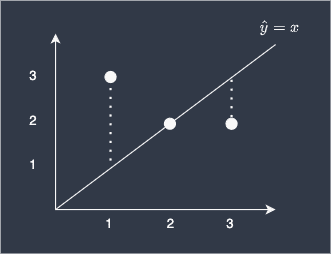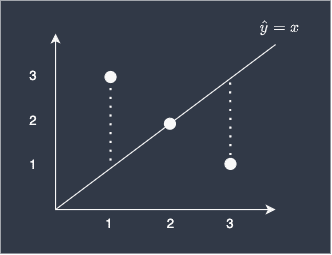search
Search
Unlock 100+ guides
search toc
close
account_circle
Profile
exit_to_app
Sign out
search
keyboard_voice
close
Searching Tips
Search for a recipe:
"Creating a table in MySQL"
Search for an API documentation: "@append"
Search for code: "!dataframe"
Apply a tag filter: "#python"
Useful Shortcuts
/ to open search panel
Esc to close search panel
to navigate between search results
d to clear all current filters
Enter to expand content previewDoc SearchCode Search BetaSORRY NOTHING FOUND!
mic
Start speaking...Voice search is only supported in Safari and Chrome.
Shrink
Navigate to
check_circle
Mark as learned
thumb_up
0
thumb_down
0
chat_bubble_outline
0
Comment
auto_stories Bi-column layout
settings

# Comprehensive Guide on Mean Absolute Error (MAE)

schedule Aug 11, 2023
Last updated
local_offer
Machine Learning
Tags
mode_heat
Master the mathematics behind data science with 100+ top-tier guides
Start your free 7-days trial now!

# What is mean absolute error (MAE)?

Mean absolute error, or MAE, measures the performance of a regression model. The mean absolute error is defined as the average of the all absolute differences between true and predicted values:

$$\mathrm{MAE}=\frac{1}{n}\sum^{n-1}_{i=0}(|y_i-\hat{y}_i|)$$

Where:

• $n$ is the number of predicted values

• $y_i$ is the actual true value of the $i$-th data

• $\hat{y}_i$ is the predicted value of the $i$-th data

A model with a high value of MAE means that its performance is subpar, while one a MAE of zero would indicate a perfect model without any error in its predictions.

# Simple example to calculate mean absolute error (MAE)

Suppose we have three data points (1,3), (2,2) and (3,2). In order to predict the y-value given the x-value, we have built a simple linear model $\hat{y}=x$, as shown in the diagram below:To get a measure of how good our model performs, we can compute the MAE like so:

\begin{align*} \mathrm{MAE}&=\frac{1}{3}\left(|3-1|+|2-2|+|2-3|\right)\\ &=\frac{1}{3}\left(2+0+1\right)\\ &=1 \end{align*}

This means that our predictions are off by one on average.

# Why do we take the absolute value?

The reason is that the absolute value prevents positive and negative differences from cancelling each other out. As an example, consider the following example:Suppose we computed the MAE without taking the absolute value:

\begin{align*} \mathrm{MAE'}&=\frac{1}{3}\Big[(1-3)+(2-2)+(3-2)\Big]\\ &=\frac{1}{3}\left(-2+0+2\right)\\ &=0 \end{align*}

As you can see, the negative and positive difference of the first and third data points have cancelled each other out. As a result, we end up with a MAE' of 0, which is obviously misleading because we know that our model is not performing all that well. In order to avoid negative and positive differences to cancel each other out, MAE takes the absolute value of the difference.

# Why isn't mean absolute error (MAE) used as a cost function?

Most machine learning models "learn" by minimising the cost function. The mean absolute error is often not chosen as the cost function because the presence of the absolute value makes differentiation harder, which means the function is difficult to optimise. For this reason, mean squared error is typically chosen as the cost function to train machine learning models.

# Computing mean absolute error (MAE) using Python's Scikit-learn

To compute the mean absolute error given a list of true and predicted values:

 from sklearn.metrics import mean_absolute_errory_true = [2,6,5]y_pred = [7,4,3]mean_absolute_error(y_true, y_pred) 3.0 

## Setting multioutput

By default, multioutput='uniform_average', which returns a the global mean absolute error:

 y_true = [[1,2],[3,4]]y_pred = [[6,7],[9,8]]mean_absolute_error(y_true, y_pred) 5.0 
thumb_up
thumb_down
Comment
Citation
Ask a question or leave a feedback...
thumb_up
0
thumb_down
0
chat_bubble_outline
0
settings
Enjoy our search
Hit / to insta-search docs and recipes!